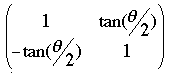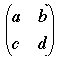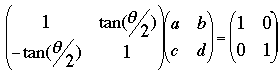SEARCH HOMEMath Central Quandaries & QueriesI'm supposed to solve ( 1 tan θ/2) ( 1 tan θ/2) -1 (-tan θ/2 1 ) (-tan θ/2 1 ) Matrix AA-1=I StephanieHi Stephanie,

If I understand this correctly you have the matrixand you are to find its inverse. That is you want to find the matrixso thatThus the first row times the first column must be 1, that is

a + c tan(θ/2) = 1

and the second row times the first column is 0, so

-a tan(θ/2) + c = 0

This gives you two equations in two unknowns which you can solve for a and c. Likewise multiply the first row time the second column and the second row times the second column to obtain equations in b and d.

I hope this helps,
PennyMath Central is supported by the University of Regina and The Pacific Institute for the Mathematical Sciences.